# 腾讯、百度、珍爱网、中国电信、三之乐面试经历2016年9月中下旬面试了5家公司。留下一点经验给后来人。

http://blog.csdn.net/gane_cheng/article/details/54142709

# 腾讯（运营开发）

1C101001p10

## 接上，现在有一种技术，一个文件分成10个小文件和3个校验文件。这13个文件中，只要有10个是正常的，文件都能恢复成功。问文件出故障的概率。

1C13100(1p13+C113p1p12+C213p21p11+C313p31p10)

## JVM原理

HotSpot中内存被分为3个代：年轻代（young generation），年老代（old generation），持久代（permanent generation）。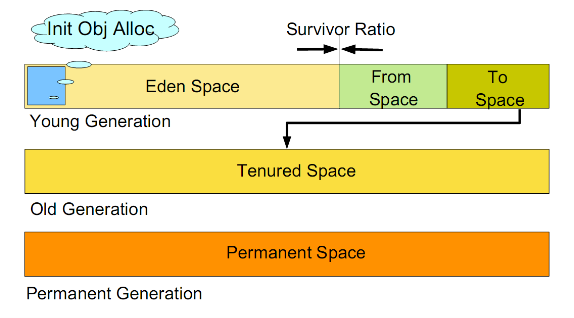## 观察者模式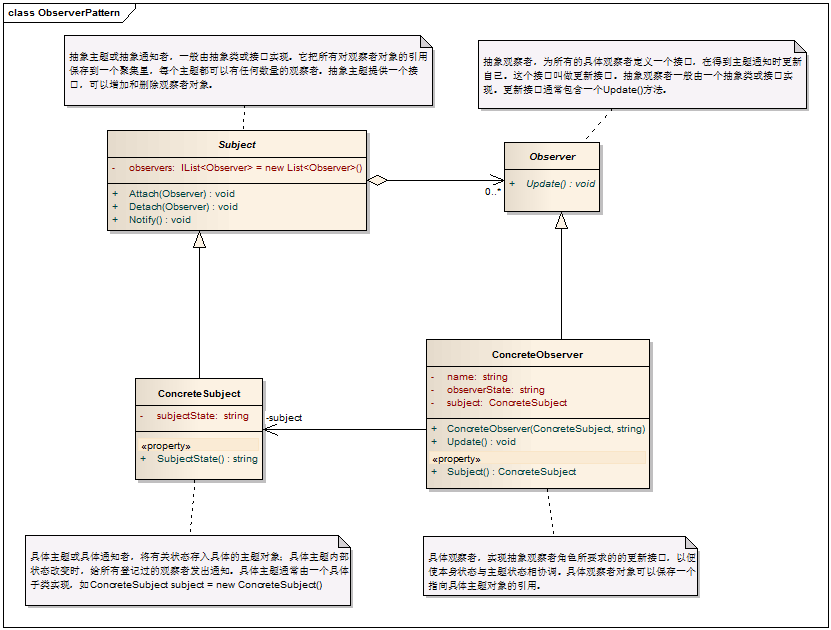# 百度（运维开发）

## 写二分查找算法

public static int rank(int goal, int[] data)
{
int start = 0;
int end = data.length - 1;
while (start <= end)
{
int mid = start + (end - start) / 2;
if (goal<data[mid])
{
end=mid-1;
}else if (goal>data[mid])
{
start=mid+1;
}else
{
return mid;
}
}

return -1;
}  

## TCP协议三次握手,四次挥手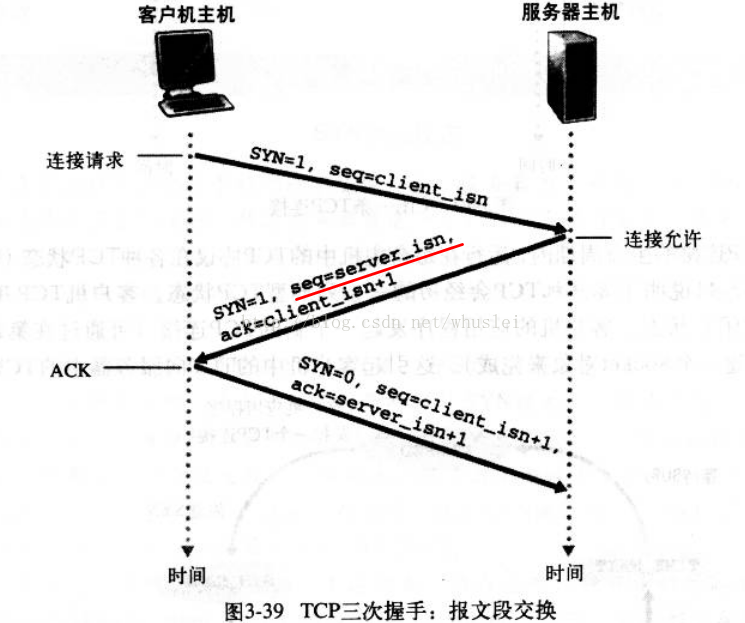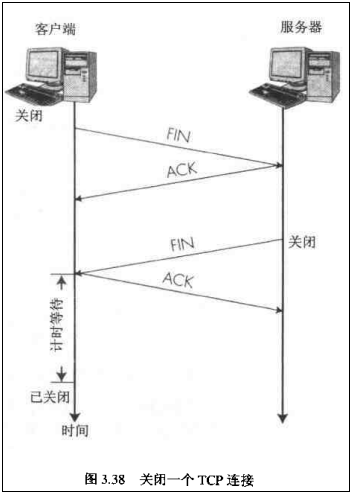## HashTable，HashMap，ConcurrentHashMap的区别。从底层实现上分析。

HashMap没有同步锁，HashTable对整个Hash表加锁，ConcurrentHashMap分段加锁。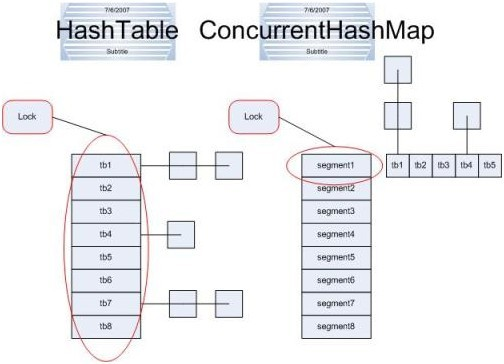## MapReduce的原理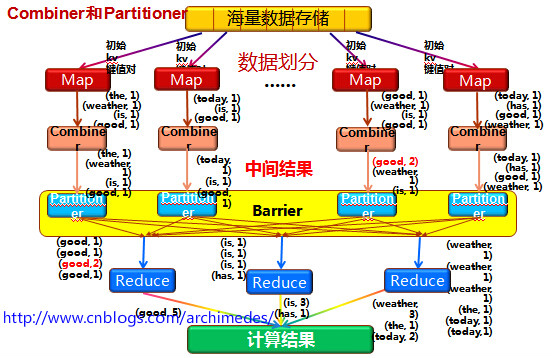## 归并排序

public static int[] sort(int[] nums, int low, int high)
{
int mid = (low + high) / 2;
if (low < high)
{
// 左边
sort(nums, low, mid);
// 右边
sort(nums, mid + 1, high);
// 左右归并
merge(nums, low, mid, high);
}
return nums;
}

public static void merge(int[] nums, int low, int mid, int high)
{
int[] temp = new int[high - low + 1];
int i = low;// 左指针
int j = mid + 1;// 右指针
int k = 0;

// 把较小的数先移到新数组中
while (i <= mid && j <= high)
{
if (nums[i] < nums[j])
{
temp[k++] = nums[i++];
}
else
{
temp[k++] = nums[j++];
}
}

// 把左边剩余的数移入数组
while (i <= mid)
{
temp[k++] = nums[i++];
}

// 把右边边剩余的数移入数组
while (j <= high)
{
temp[k++] = nums[j++];
}

// 把新数组中的数覆盖nums数组
for (int k2 = 0; k2 < temp.length; k2++)
{
nums[k2 + low] = temp[k2];
}
}

## 希尔排序

        int[] a = { 49, 38, 65, 97, 76, 13, 27, 49, 78, 34, 12, 64, 1 };
System.out.println("排序之前：");
for (int i = 0; i < a.length; i++)
{
System.out.print(a[i] + " ");
}
// 希尔排序
int d = a.length;
while (true)
{
d = d / 2;
for (int x = 0; x < d; x++)
{
for (int i = x + d; i < a.length; i = i + d)
{
int temp = a[i];
int j;
for (j = i - d; j >= 0 && a[j] > temp; j = j - d)
{
a[j + d] = a[j];
}
a[j + d] = temp;
}
}
if (d == 1)
{
break;
}
}
System.out.println();
System.out.println("排序之后：");
for (int i = 0; i < a.length; i++)
{
System.out.print(a[i] + " ");
}

## 快速排序

    private static void quick(int[] a)
{
if (a.length > 0)
{
quickSort(a, 0, a.length - 1);
}
}

private static void quickSort(int[] a, int low, int high)
{
if (low < high)
{ // 如果不加这个判断递归会无法退出导致堆栈溢出异常
int middle = getMiddle(a, low, high);
quickSort(a, 0, middle - 1);
quickSort(a, middle + 1, high);
}
}

private static int getMiddle(int[] a, int low, int high)
{
int temp = a[low];// 基准元素
while (low < high)
{
// 找到比基准元素小的元素位置
while (low < high && a[high] >= temp)
{
high--;
}
a[low] = a[high];
while (low < high && a[low] <= temp)
{
low++;
}
a[high] = a[low];
}
a[low] = temp;
return low;
}

## 给栈增加一个方法,返回栈中第二大的元素,时间复杂度为O(1)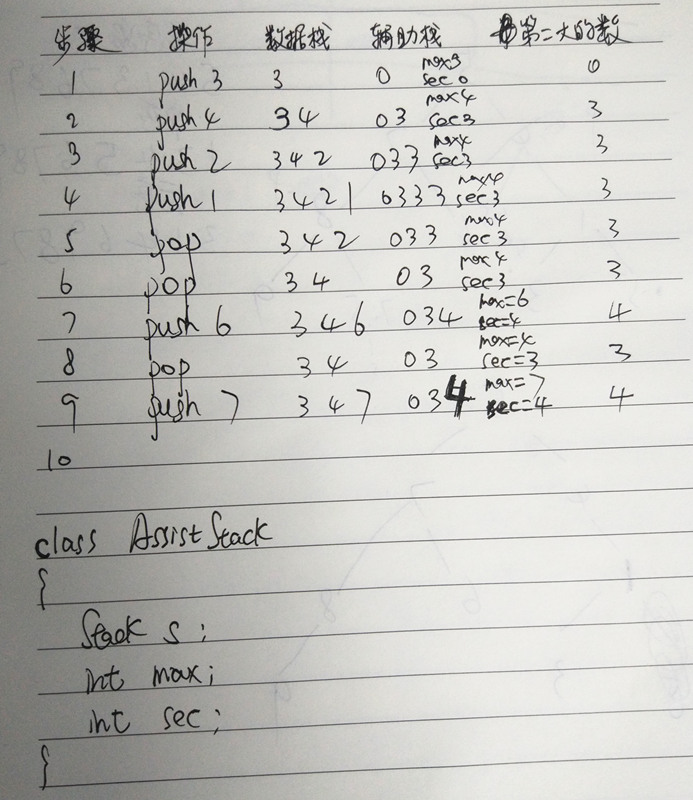# 珍爱网（Java开发）

## spring两大特性的理解及底层实现

AOP面向切面编程，可以实现事务，权限，日志等功能的统一实现，使代码进一步的解耦。底层使用代理模式实现的。

## HashTable，HashMap，ConcurrentHashMap的底层区别？

HashMap没有同步锁，HashTable对整个Hash表加锁，ConcurrentHashMap分段加锁。

## HashMap中key可不可以为null，存在哪里？

    final Entry<K,V> getEntry(Object key) {
if (size == 0) {
return null;
}

//重点是这一句
int hash = (key == null) ? 0 : hash(key);

for (Entry<K,V> e = table[indexFor(hash, table.length)];
e != null;
e = e.next) {
Object k;
if (e.hash == hash &&
((k = e.key) == key || (key != null && key.equals(k))))
return e;
}
return null;
}

public V put(K key, V value) {
if (table == EMPTY_TABLE) {
inflateTable(threshold);
}
if (key == null)
return putForNullKey(value); //重点这一句
int hash = hash(key);
int i = indexFor(hash, table.length);
for (Entry<K,V> e = table[i]; e != null; e = e.next) {
Object k;
if (e.hash == hash && ((k = e.key) == key || key.equals(k))) {
V oldValue = e.value;
e.value = value;
e.recordAccess(this);
return oldValue;
}
}

modCount++;
addEntry(hash, key, value, i);
return null;
}

private V putForNullKey(V value) {
for (Entry<K,V> e = table; e != null; e = e.next) {
if (e.key == null) {
V oldValue = e.value;
e.value = value;
e.recordAccess(this);
return oldValue;
}
}
modCount++;
addEntry(0, null, value, 0);//重点这一句
return null;
}

# 三之乐（Java开发）

9月14日笔试，9月20日拿到Java开发的Offer。总共有1次笔试+一次电话面试+三次现场面试。下面记录一下我的经历，希望能为后来人提供经验。

## ①笔试

9月14日下午2:30去的公司。HR为我准备了一套试卷，要求在1小时内做完。难度不大，都是基础题，有几道记忆比较深的题，记录一下。

1.给出一段英文句子。要求将英文句子逆序输出来。例如“I’m a boy, she is a girl.”输出”. girl a is she , boy a I’m”。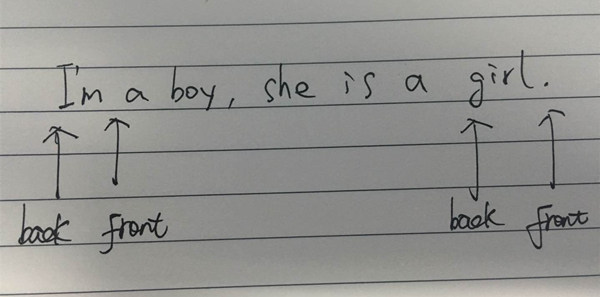2.给定一个数据库的表Test，表的内容如图所示。

id class score
1 one 91
2 one 92
3 two 93
4 two 94

one two
2 2

select class ,count(id) from test group by class

class count(id)
one 2
two 2

select distinct
count(case class when 'one' then 1 else 0 end) one,
count(case class when 'two' then 1 else 0 end) two
from test
group by class

3.专业名词解释。

# 结束语